Recombination Parameters

 Remarks Referens 4H-SiC. Pure n-type material The longest lifetime of holes tp ~= 6.0 x 10-7 c 300 K Diffusion length Lp = (Dp x tp)1/2 ~= 12 μm c 300 K 4H-SiC. Pure p-type material The longest lifetime of electrons tn ~= 10-9 c 300 K Diffusion length Ln = (Dn x tn)1/2 ~= 1.5 μm 300 K 4H-SiC Surface Recombinaton Velocity 103 - 105 300 K Galeskas et al. (1997); Grivickas et al. (1997); Neudeck & Fazi (1997); Kimoto et al. (1999) 4H-SiC Radiative recombination coefficient B 1.5 x 10-12 cm3/s 300 K, estimation Galeskas et al. (1997) 4H-SiC Auger coefficient Cn 5.0 x 10-31 cm6/s 300 K Galeskas et al. (1997) Auger coefficient Cp 2.0 x 10-31 cm6/s 300 K Galeskas et al. (1997) Auger coefficient C = Cn + Cp 7.0 x 10-31 cm6/s 300 K Galeskas et al. (1997) 6H-SiC. Pure n-type material The longest lifetime of holes tp ~= 4.5 x 10-7 c 300 K Diffusion length Lp = (Dp x tp)1/2 ~= 10 μm c 300 K 6H-SiC. Pure p-type material The longest lifetime of electrons tn ~= 10-9 c 300 K Diffusion length Ln = (Dn x tn)1/2 ~= 1 μm 300 K 6H-SiC Surface Recombinaton Velocity 104 - 105 300 K 6H-SiC Radiative recombination coefficient B ~=1.5 x 10-12 cm3/s 300 K. Galeskas et al. (1997) The values measured in 4H-SiC can be used for estimations. 6H-SiC Auger coefficient Cn ~=5.0 x 10-31 cm6/s 300 K Auger coefficient Cp ~=2.0 x 10-31 cm6/s 300 K Auger coefficient C = Cn + Cp ~=7.0 x 10-31 cm6/s 300 K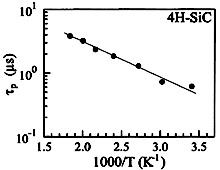4H-SiC. Hole lifetime tp vs. reciprocal temperature measured in the base of a high-voltage 4H-SiC rectifier diode. Ivanov et al. (1999)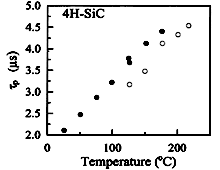4H-SiC. Hole lifetime tp vs. reciprocal temperature measured using the photoluminescence decay technique. Kordina et al. (1996)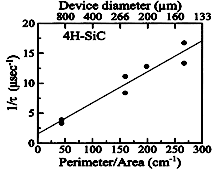4H-SiC. Reciprocal effective hole lifetime 1/t vs. perimeter-to-area P/A ratio for 4H-SiC p-n structure. The slope of the dependence defines the surface recombination velocity according to the equation 1/t= 1/tp + s x (A/P), where tp is the volume hole lifetime. Neudeck & Fazi (1997)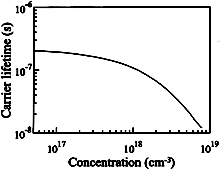4H-SiC. The carriers lifetime vs. carrier density. Solid curve is calculated to fit experimental data: t = 2.6 x 10-7 s; B = 1.5 x 10-12 cm3/s; C = 7 x 10-31 cm6/s. Galeskas et al. (1997)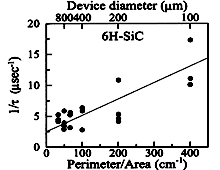6H-SiC. Reciprocal effective hole lifetime 1/t vs. perimeter-to-area P/A ratio for 6H-SiC p-n structure. The slope of the dependence defines the surface recombination velocity according to the equation 1/t= 1/tp + s x (A/P), where tp is the volume hole lifetime. Kimoto et al. (1999)